Home / Tutorials / Arduino Tutorial / How to Use ADXL345 Accelerometer with Arduino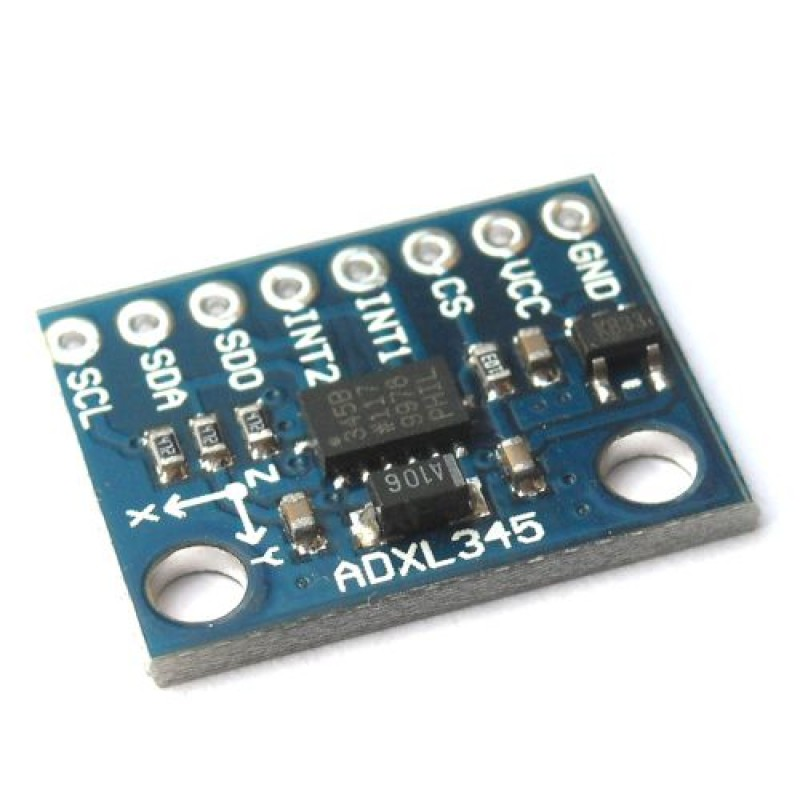# How to Use ADXL345 Accelerometer with Arduino

The ADXL345 is an integrated circuit that measures acceleration in 3 axes. Like most sensors, this device comes with an easy-to-use breakout board and is usable through SPI or I2C. This tutorial aims to show how you can interface this accelerometer with an Arduino.

In reality, the ADXL345 is capable of measuring acceleration forces in the x, y and z axes.Acceleration force is the force that causes an object to move. If an object is at rest, only the force of gravity is acting on it. Hence, the ADXL345 gives acceleration forces in g units, g being the acceleration due to gravity equal to 9.8 m/s2.

The ADXL345 can measure acceleration in as much as ±2g with an option to change the maximum to ±4g, ±8g and ±16g. The acceleration forces can be static (due to gravity only) or dynamic (due to motion and gravity).

A more in-depth look at the ADXL345 is accessible via its datasheet:

[wpdm_package id=’3554′]

This tutorial will focus on the ADXL345 breakout board as shown:If you own a different board, rest assure that the sketches in this tutorial will still work as long as the correct wiring is followed.

### Setting Up Arduino IDE

First, we need the ADXL345 Arduino library. Open Arduino IDE (ver. 1.8.9) then go to Tools > Manage Libraries…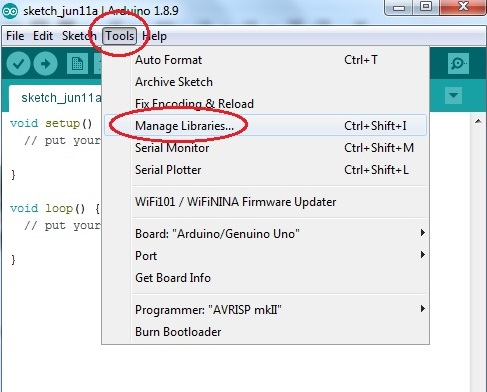The Library Manager now appears. On the search field, type “Adafruit ADXL345”, press enter and the library should appear.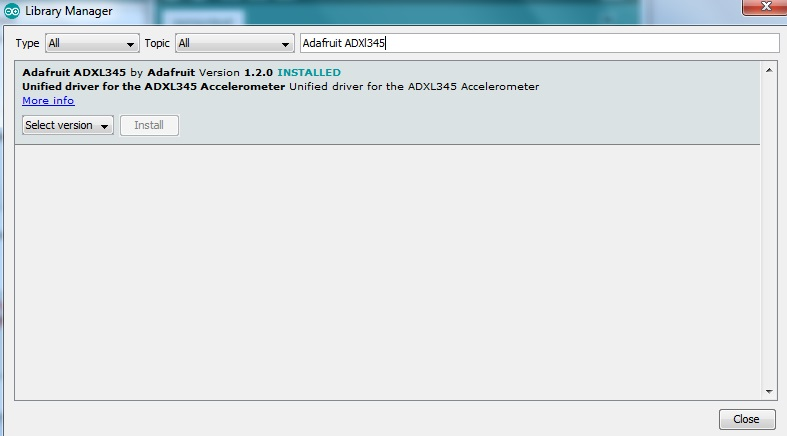We also need to install the Unified Sensor library: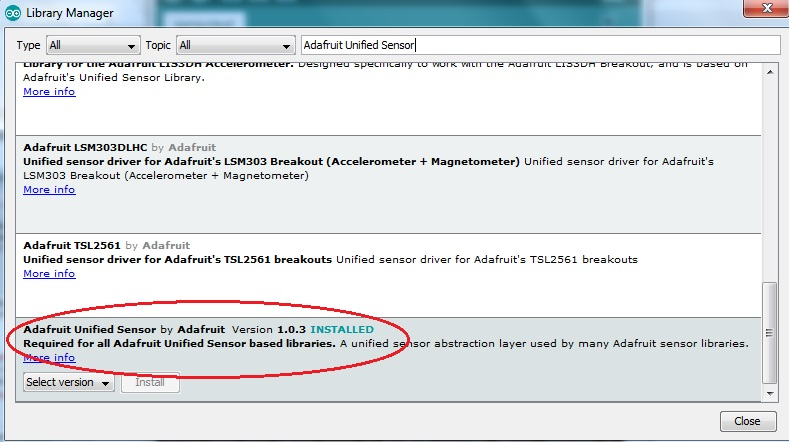### Using the ADXL345 with Arduino

After installation of the libraries, go to Files > Examples > Adafruit ADXL345 > sensortest. The example sketch is as shown:

To use this sketch, follow this wiring diagram: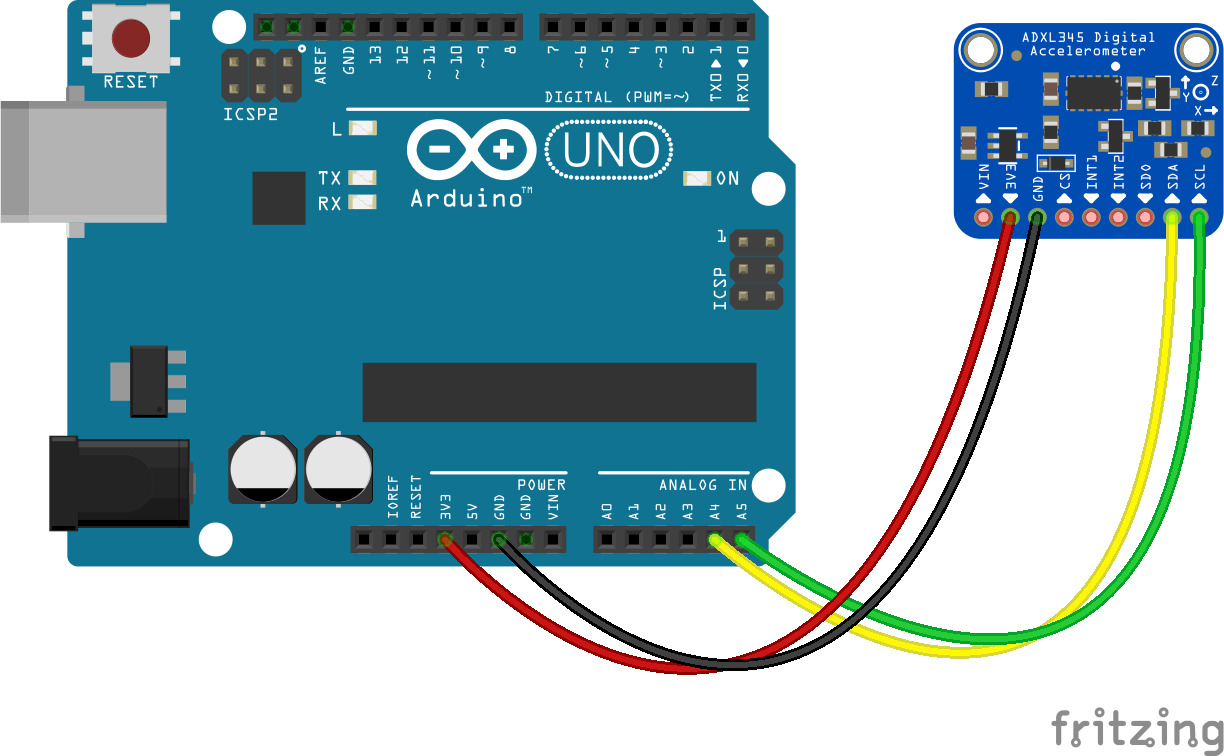For other boards, make sure that this connection is followed:

3.3V VDD
GND GND
A4 SDA/SDI
A5 SCL/SCLK

Upload the sketch to your Arduino and open Serial Monitor. The acceleration values is now visible: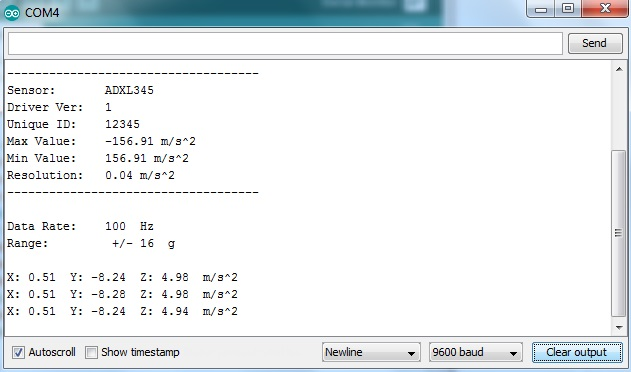The library converts the output to m/s2 instead of g units.

### Explaining the Sketch

#### Display Sensor Details

The sensortest sketch comes with three functions beside the usual setup() and loop(). The first function is the displaySensorDetails() function:

This function displays the sensor type, maximum and minimum values, resolution and other information about the ADXL345. This function is responsible for this part of the output on the serial monitor:

The next function is getDataRate():

This function returns the current data rate of the ADXL345. This also gives us the usable data rates from the slowest (10 Hz) to the fastest (3200 Hz). The datasheet tells us about data rate selection:

An output data rate should be selected that is appropriate for the communication protocol and frequency selected. Selecting too high of an output data rate with a low communication speed results in samples being discarded.

This means rate selection is dependent on the speed of the microcontroller device. The Arduino UNO supports high-speed I2C mode which has a speed of 3.4 MHz so it will not have trouble at higher data rates.

To change data rate, use the function setDataRate(datarate). The datarate can be any of the following:

For example, to change the data rate to 400 Hz, place this inside setup(), after accel.begin():

#### Range Value

The last function is the displayRange() function:

This prints out the current range of the ADXL345. If ±2g is selected, the accelerometer can now detect acceleration up to ±19.6 m/s2 (2 * 9.8). The default value is ±16g which is equivalent ±156.8 m/s2.

To change the range, use the setRange(range) function. The range can be any of the following:

For example, the following changes the range to ±2g:

Make sure that the above code is after the accel.begin() function inside setup().

### Demonstration Video

Here’s a video showing the output of the sketch:

### Explaining the Output

As mentioned, the ADXL345 measures acceleration forces. If the sensor is at rest, only the gravitational force is acting on it. If it is moving, then it will read the force that is applied.

At rest and with the sensor placed flat as pictured, we expect zero (or almost zero) values in both x and y axes, and a value equal to g for the z axes.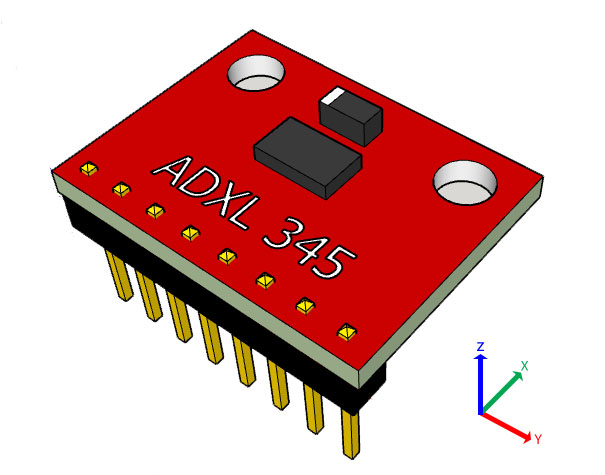Tilt the board as shown and the sensor is now going against gravity in the x axis, hence we expect a non-zero value on x.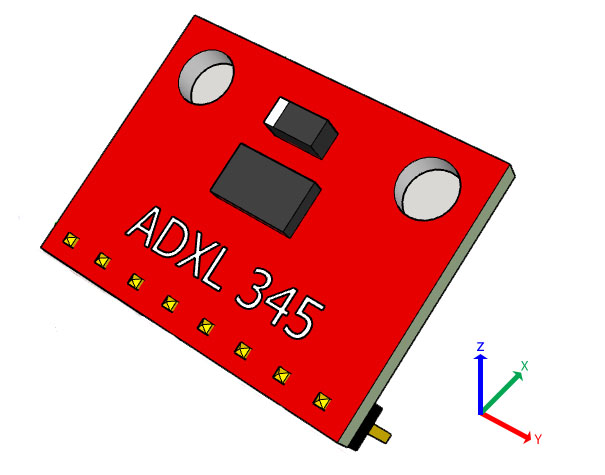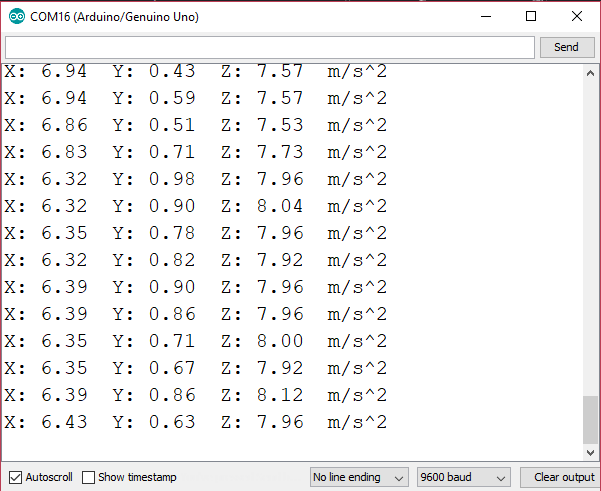Tilt the board as shown and the sensor is now going against gravity in the y axis, and a non-zero y value is now read.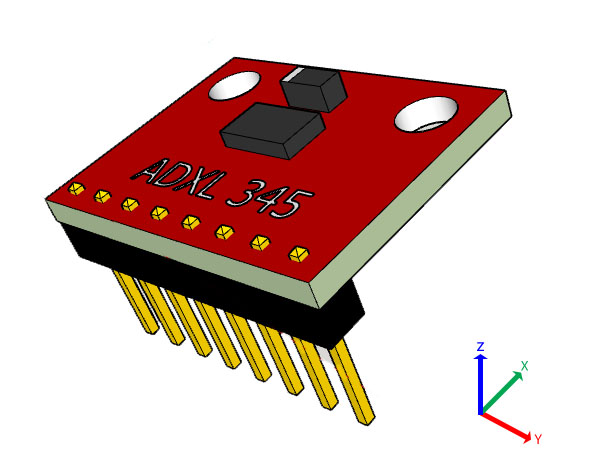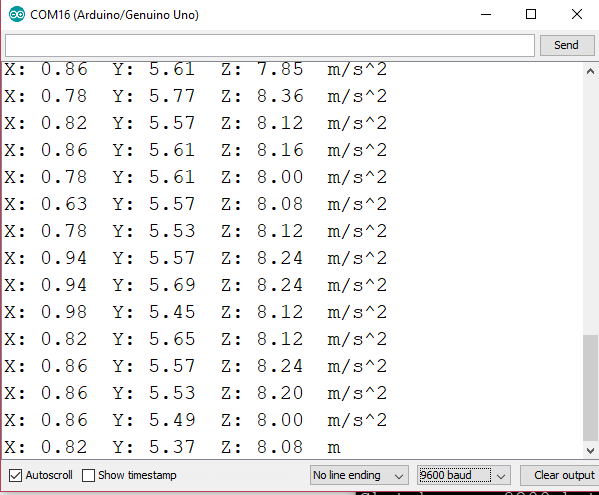Move the sensor in a certain direction and it will give the acceleration due to the applied force and gravity!

If you find this tutorial helpful, kindly drop a comment below!

## MAX30102: An Improved Heart Rate Sensor for Arduino

Have you read the MAX30100 tutorial and are still having trouble making that chip work? …